# 3.11.13-Setup & Solve The legs of an isosceles right triangle increase in length at a rate...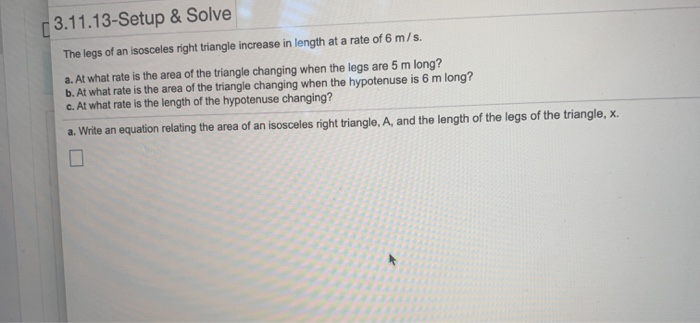3.11.13-Setup & Solve The legs of an isosceles right triangle increase in length at a rate of 6 m/s. a. At what rate is the area of the triangle changing when the legs are 5 m long? b. At what rate is the area of the triangle changing when the hypotenuse is 6 m long? c. At what rate is the length of the hypotenuse changing? a. Write an equation relating the area of an isosceles right triangle, A, and the length of the legs of the triangle, X.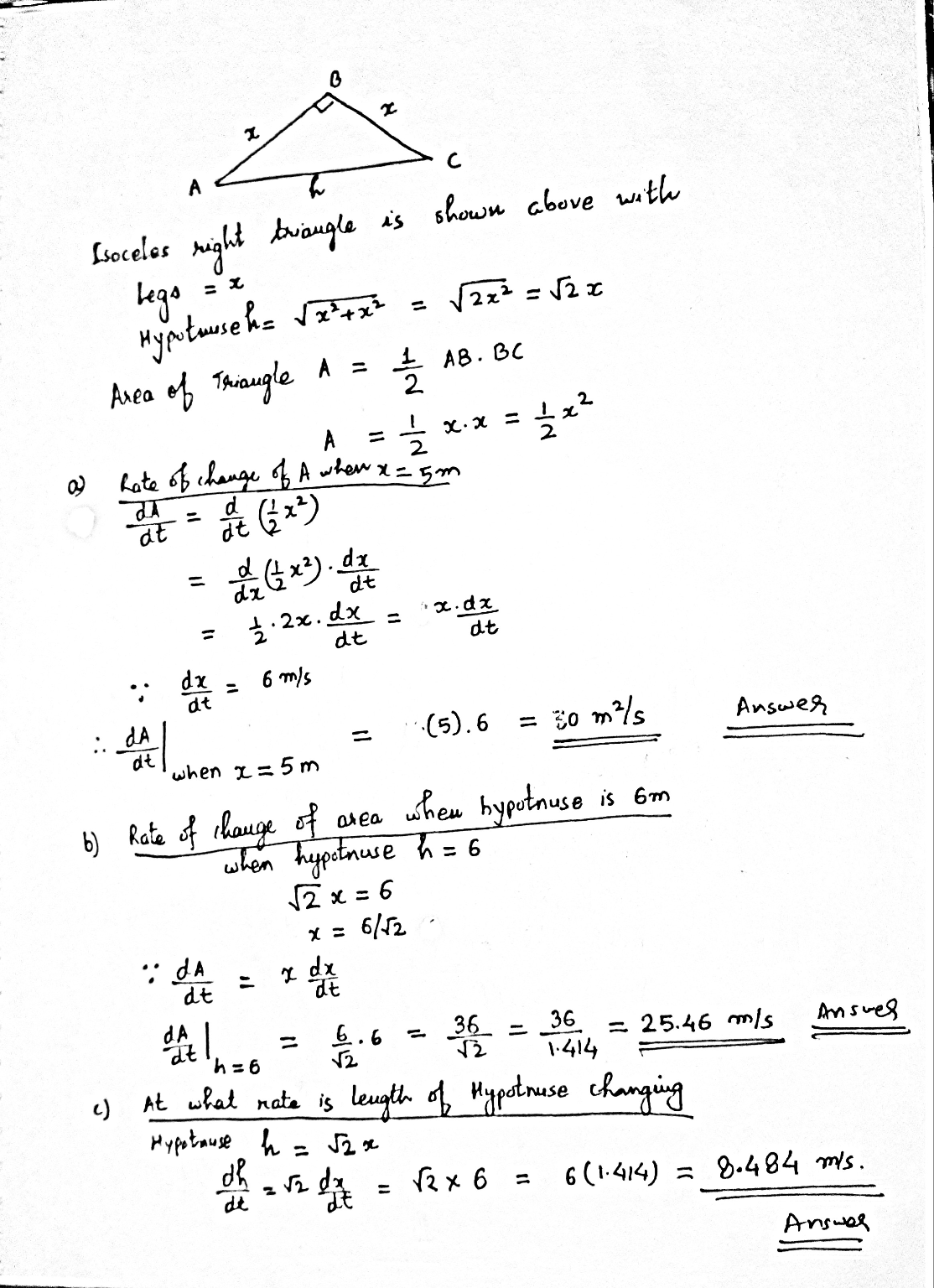#### Earn Coin

Coins can be redeemed for fabulous gifts.

Similar Homework Help Questions
• ### Find the length of the hypotenuse of an isosceles right triangle whose legs are 1 unit in length

Find the length of the hypotenuse of an isosceles right triangle whose legs are 1 unit in length.

• ### hw question help please!! 5. (8 pts) An isosceles right triangle is as shown in the...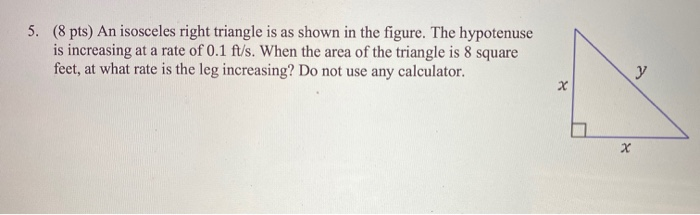hw question help please!! 5. (8 pts) An isosceles right triangle is as shown in the figure. The hypotenuse is increasing at a rate of 0.1 ft/s. When the area of the triangle is 8 square feet, at what rate is the leg increasing? Do not use any calculator. y x X

• ### The length of one of the equal legs of an isosceles triangle is 8 cm less than 4 times the length of the base

1.The length of one of the equal legs of an isosceles triangle is 8 cm less than 4 times the length of the base. If the perimeter is 29 cm, find the length of one of the equal legs.A)4 cmB)5 cmC)11 cmD)12 cm answers4x-8= 4(5)-8= 20-8= 122.3x - 2y = 6answerX=4 and Y=33*4=12x = 2/3y + 23.solve. -3(x+1)=2(x-8)+3ANSWERX=2

• ### The Pythagorean Theorem for right triangles states that if a and b are the lengths of the legs of a right triangle and c is the length of the hypotenuse, then a2 C b2 D c2

The Pythagorean Theorem for right triangles states that if a and b are thelengths of the legs of a right triangle and c is the length of the hypotenuse,then a2 C b2 D c2

• ### Let a and b be the lengths of the legs of a right triangle, and c...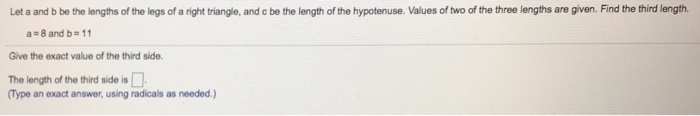Let a and b be the lengths of the legs of a right triangle, and c be the length of the hypotenuse. Values of two of the three lengths are given. Find the third length. a 8 and b 11 Give the exact value of the third side. The length of the third side is Type an exact answer, using radicals as needed.)

• ### The coordinates of the vertices of a right triangle are (0,0), (5,0),and (0,3). Which of the following statements is true?

A) The midpoints of the legs are (0,1.5) and (2.5,0) B) The shorter of the two legs of the triangle is positioned on the x- axis C) The vertex of the right angle is located at (5,0) D) The length of the hypotenuse is 5

• ### Find the length of the missing side of the right triangle. Round to three decimal places,...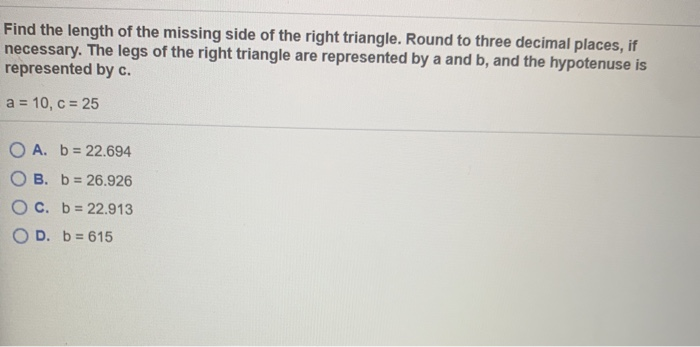Find the length of the missing side of the right triangle. Round to three decimal places, if necessary. The legs of the right triangle are represented by a and b, and the hypotenuse is represented by c. a = 10, C = 25 O A. b = 22.694 OB. b = 26.926 c. b = 22.913 OD. b = 615

• ### the Pythagorean theorem to find the length of the unknown side of a right triangle, where...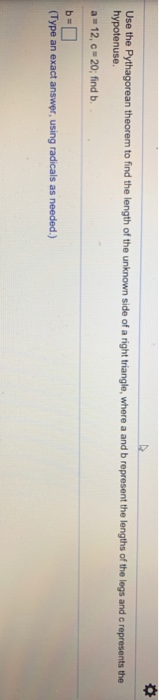the Pythagorean theorem to find the length of the unknown side of a right triangle, where a and b represent the lengths of the legs and c represents the hypotenuse. a 12, c 20, find b (Type an exact answer using radicals as needed.)

• ### X + 9 The length of the shortest side of the isosceles triangle is 6 inches....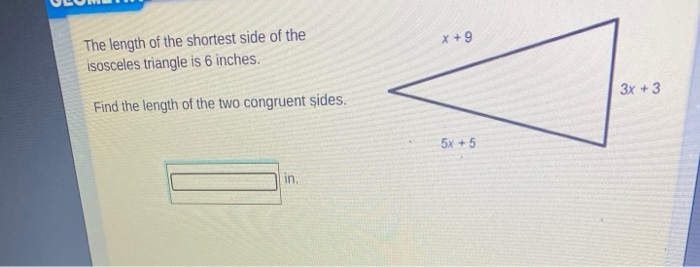X + 9 The length of the shortest side of the isosceles triangle is 6 inches. 3x + 3 Find the length of the two congruent sides. 5x + 5 in GEOMETRY IA If x = 35, is the triangle acute, right, or obtuse? 3(x - 15) 2(x - 5) X + 25

• ### 6. The sides of a right triangle are measured to be a = 7.80 ± 0.02...

6. The sides of a right triangle are measured to be a = 7.80 ± 0.02 cm and b = 6.40 ± 0.02 cm. Determine the length of the hypotenuse of the triangle (given by ?^2 +?^2 = ?^2) and the uncertainty in the length. (Hint: you need to use the derivative equation to find the uncertainty.) 7. If you drive 146 miles in a time of 3.25 hours, what is your average speed in km/h and m/s? How long...